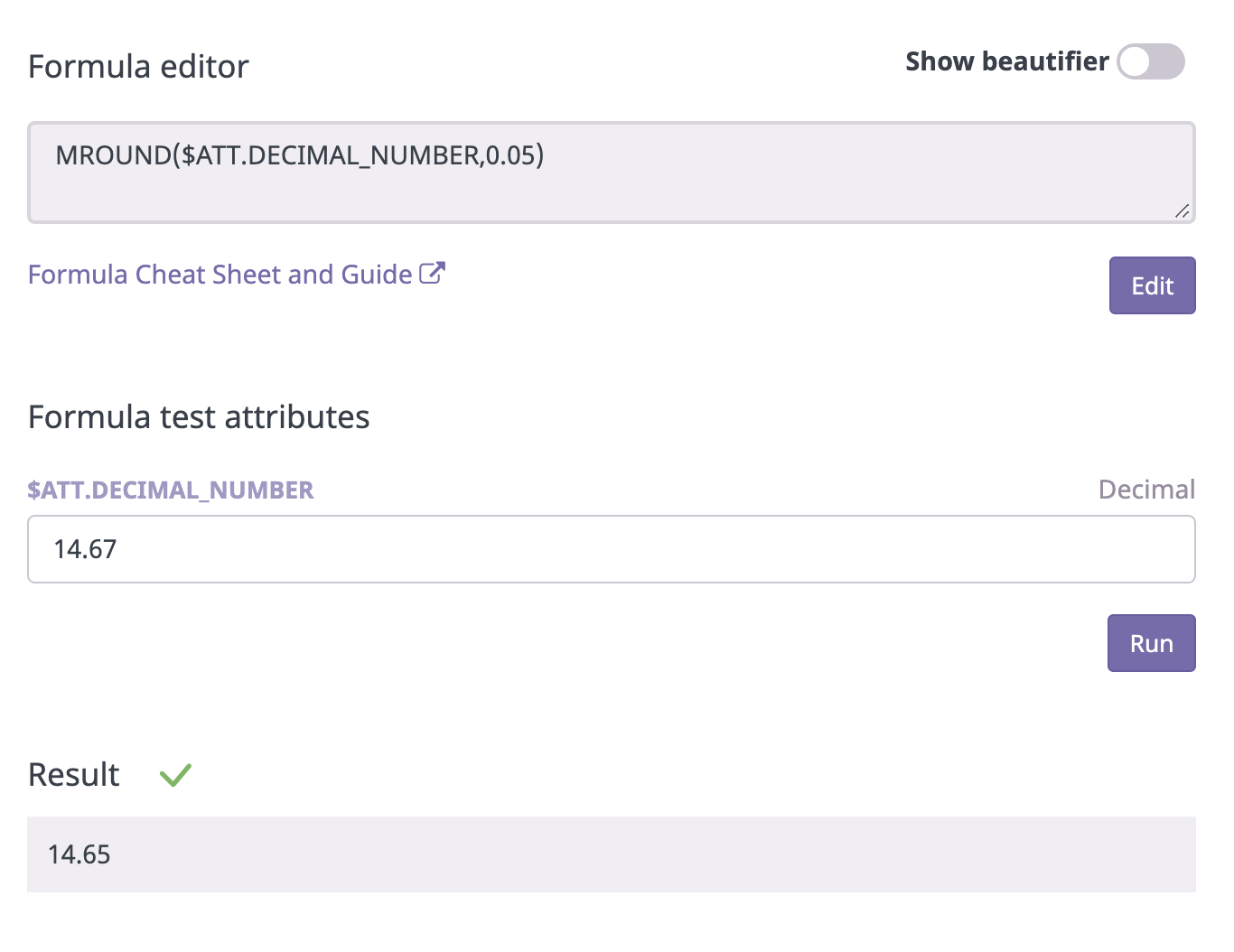# MROUND Operation

## How to use the MROUND operation for Computed Attributes

### Definition

The MROUND operation rounds a number to the nearest multiple.

• This includes both integer and decimal multiples

MROUND(47,10)

Result:
50

### Syntax Guide

MROUND(value,factor)

value - The number to round to the nearest multiple of another

factor - The number to whose multiples value will be rounded### Formulas In Use

#### Currency Conversion and Rounding

Converts a currency with a fixed exchange rate and rounds the result to always end on the same decimal (eg. .95)

Instead of converting with a fixed conversion rate where you get odd numbers, like for example:

100 dkk / 7,45 =13.42 EUR

Then this script can convert the odd calculated number 13,42 a "prettier" number

100 DKK --> 12.95 EUR

Formula:

MINUS(MROUND(DIVIDE(\$ATT.PRICE_DKK,7.45),1),0.05)